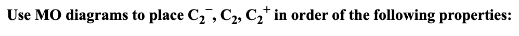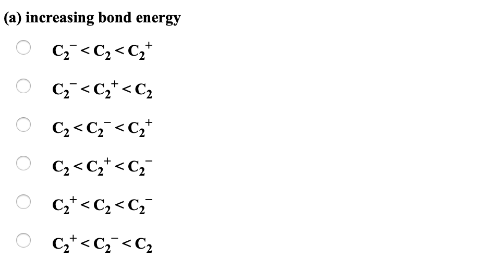# Problem: Use MO diagrams to place C2^- ,C2, C2^+ in order of the following properties: (a) Increasing bond energy a) C2^- &lt; C2 &lt; C2^+ b) C2^- &lt; C2^+ &lt; C2 c) C2 &lt; C2^- &lt; C2^+ d) C2 &lt; C2^+ &lt; C2^- e) C2^+ &lt; C2 &lt; C2^- f) C2^+ &lt; C2^- &lt; C2

###### FREE Expert Solution
87% (414 ratings)###### Problem Details

Use MO diagrams to place C2^- ,C2, C2^+ in order of the following properties: (a) Increasing bond energy a) C2^- < C2 < C2^+ b) C2^- < C2^+ < C2 c) C2 < C2^- < C2^+ d) C2 < C2^+ < C2^- e) C2^+ < C2 < C2^- f) C2^+ < C2^- < C2